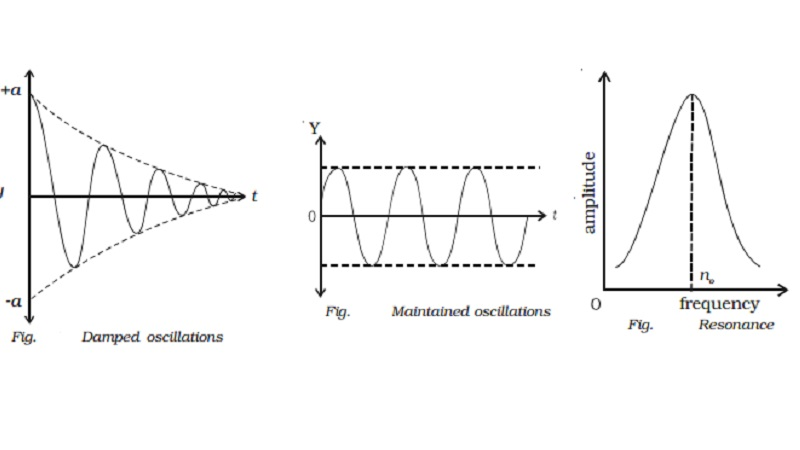Home | | Physics | | Physics | Types of oscillations

# Types of oscillations(i)Free oscillations ii. Damped oscillations (iii ) Maintained oscillations (iv ) Forced oscillations (v ) Resonance

Types of oscillations

There are three main types of oscillations.

(i)Free oscillations

When a body vibrates with its own natural frequency, it is said to execute free oscillations. The frequency of oscillations depends on the inertial factor and spring factor, which is given by,

n=1/2π root(k/m)

Examples

(i)                (I) Vibrations of tuning fork

(ii)              (ii) Vibrations in a stretched string

(iii)           (iii) Oscillations of simple pendulum

(iv)           (iv) Air blown gently across the mouth of a bottle.

ii. Damped oscillations

Most of the oscillations in air or in any medium are damped. When an oscillation occurs, some kind of damping force may arise due to friction or air resistance offered by the medium. So, a part of the energy is dissipated in overcoming the resistive force. Consequently, the amplitude of oscillation decreases with time and finally becomes zero. Such oscillations are called damped oscillations (Fig.).Examples :

i)                   The oscillations of a pendulum

ii)                Electromagnetic damping in galvanometer (oscillations of a coil in galvanometer)

iii)              Electromagnetic oscillations in tank circuit

(iii ) Maintained oscillations

The amplitude of an oscillating system can be made constant by feeding some energy to the system. If an energy is fed to the system to compensate the energy it has lost, the amplitude will be a constant. Such oscillations are called maintained oscillations (Fig.).Example :

A swing to which energy is fed continuously to maintain amplitude of oscillation.

(iv ) Forced oscillations

When a vibrating body is maintained in the state of vibration by a periodic force of frequency (n) other than its natural frequency of the body, the vibrations are called forced vibrations. The external force is driver and body is driven.

The body is forced to vibrate with an external periodic force. The amplitude of forced vibration is determined by the difference between the frequencies of the driver and the driven. The larger the frequency difference, smaller will be the amplitude of the forced oscillations.

Examples :

(i)                Sound boards of stringed instruments execute forced vibration,

(ii)             Press the stem of vibrating tuning fork, against tabla. The tabla suffers forced vibration.

(v ) Resonance

In the case of forced vibration, if the frequency difference is small,

the amplitude will be large (Fig.). Ultimately when the two frequencies are same, amplitude becomes maximum.This is a special case of forced vibration..If the frequency of the external periodic force is equal to the natural frequency of oscillation of the system, then the amplitude of oscillation will be large and this is known as resonanc.

(i)                Using resonance, frequency of a given tuning fork is determined with a sonometer.

(ii)             In radio and television, using tank circuit, required frequency can be obtained.

(i) Resonance can cause disaster in an earthquake, if the natural frequency of the building matches the frequency of the periodic oscillations present in the Earth. The building begins to oscillate with large amplitude thus leading to a collapse.

(ii)                   A singer maintaining a note at a resonant frequency of a glass, can cause it to shatter into pieces

Study Material, Lecturing Notes, Assignment, Reference, Wiki description explanation, brief detail

Related Topics